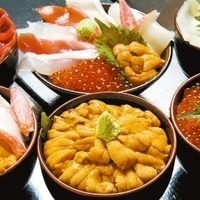マルカン漁業部 海がき 本店

Umigaki

• 信用卡
• 吸烟
• 海鲜料理,居酒屋,生鱼片 / 海鲜
• 晚餐 4,000 日元
• 0138-27-4488 (+81-138-27-4488)
• 17:00～24:00(L.O.23:15, 酒水L.O.23:30)
• 函馆(北海道)
JR函馆本线 函馆站 步行3分钟

*这里描述的食材信息是代表性的，并非所有食材信息。使用其他食材的时候也有。此外，根据季节变化或当天采购情况食材也会有变动，请谅解。

*照片仅供参考。

*显示价格仅供参考，实际售价依各店为准。

* 金额为日元（JPY）

【函館名物】活いか刺し踊り漁師造り

• 食材: 乌贼
• 烹饪方法:

• 食材: 章鱼
• 烹饪方法:

キングサーモン刺し

• 食材: 帝王鲑
• 烹饪方法:

• 食材: 章鱼
• 烹饪方法:

ずわいがにのむき身

• 食材: 雪蟹
• 烹饪方法:

ミンク鯨のベーコン

• 食材: 鲸鱼肉
• 烹饪方法:

• 食材: 马肉
• 烹饪方法:

ほっけ刺し

• 食材: 远东多线鱼
• 调味料: 酱油
• 烹饪方法:

そい刺し

• 调味料: 酱油
• 烹饪方法:

• 食材: 比目鱼
• 烹饪方法:

ぶり刺し

• 食材: 鰤鱼

• 食材: 甜虾
• 烹饪方法:

• 食材: 白肉：其他
• 烹饪方法:

5种海鲜刺身的拼盘料理。

• 烹饪方法:

7种海鲜刺身的拼盘料理。

• 烹饪方法:

• 食材: 牡丹虾
• 烹饪方法:

• 食材: 乌贼
• 烹饪方法:

• 食材: 远东多线鱼
• 烹饪方法: 晒干, 烤

• 食材: 柳叶鱼
• 烹饪方法:

• 食材: 细身宽突鳕
• 烹饪方法:

• 食材: 鳕鱼
• 烹饪方法:

• 食材: 鲑鱼肚
• 烹饪方法:

つぼだい開き

• 食材: 鲷鱼
• 烹饪方法:

• 食材: 鲱鱼
• 烹饪方法:

5种串烧拼盘

5种类的肉，蔬菜等材料切成适当大小穿成串，直接用火烤制的拼盘料理。

• 食材: 芦笋, 紫苏, 青椒, 葱, 乌贼
• 烹饪方法: 串烤

• 食材: 鸡肉
• 烹饪方法: 串烤

• 食材: 猪肉
• 烹饪方法: 串烤

• 食材: 牛舌
• 烹饪方法: 串烤

ラム串

• 食材: 羊羔肉
• 烹饪方法: 串烤

• 食材: 牛里脊肉
• 烹饪方法: 串烤

• 食材: 牛横隔膜
• 烹饪方法: 串烤

ジンギスカン

• 食材: 羊肉
• 烹饪方法: 串烤

ほっけちゃんちゃん焼き

• 食材: 远东多线鱼
• 调味料: 味噌
• 烹饪方法: 蒸, 烤

• 食材: 鲑鱼
• 调味料: 味噌
• 烹饪方法: 蒸, 烤

• 食材: 乌贼
• 烹饪方法:

まぐろ山かけ

• 食材: 金枪鱼, 山药
• 烹饪方法:

盐辛鱿鱼

• 食材: 乌贼, 乌贼、章鱼、海参等。:其他
• 调味料: 食盐
• 烹饪方法:

ほや塩辛

• 食材: 海鞘
• 烹饪方法: 发酵

かにみそ（かに身付）

• 食材: 螃蟹, 蟹黄

いか沖漬けルイベ

• 食材: 乌贼
• 调味料: 酱油
• 烹饪方法: 醃, 冷冻

いくら醤油漬け

• 食材: 鲑鱼卵
• 调味料: 酱油
• 烹饪方法:

• 食材: 马铃薯, 塩辛
• 调味料: 黄油

グリーンアスパラ1本焼き

• 食材: 芦笋
• 烹饪方法:

• 食材: 长山药, 豆腐
• 烹饪方法:

• 食材: 黄瓜
• 调味料: 味噌, 蛋黃醬

• 烹饪方法:

なす焼き

• 食材: 茄子, 柴鱼片
• 调味料: 酱油, 高汤
• 烹饪方法: 切, 烤

• 调味料: 味噌
• 烹饪方法:

ポテトフライ

• 食材: 马铃薯
• 烹饪方法:

ほたてフライ

• 食材: 扇贝, 面包屑
• 烹饪方法:

ほっけフライ

炸远东多线鱼

• 食材: 远东多线鱼, 面包屑
• 烹饪方法:

かきフライ

• 食材: 牡蛎
• 烹饪方法:

• 食材:
• 烹饪方法:

げそ揚げ

• 食材: 乌贼
• 烹饪方法:

たこ揚げ

• 食材: 章鱼
• 烹饪方法:

• 食材: 葱, 豆腐
• 烹饪方法:

ざんぎ（鶏の唐揚げ）

• 食材: 鸡大腿肉
• 烹饪方法:

炸虾等炸海鲜拼盘

• 食材: 虾, 远东多线鱼, 牡蛎, 乌贼
• 烹饪方法:

ラーメンサラダ

• 食材: 中华汤面
• 烹饪方法: 混合

• 烹饪方法: 混合

かにサラダ

• 食材: 螃蟹
• 烹饪方法: 混合

たこサラダ

• 食材: 章鱼
• 烹饪方法: 混合

かに酢

醋拌螃蟹

• 食材: 螃蟹
• 调味料: 醋, 高汤
• 烹饪方法: 混合

いか・いか・いか全部丼（足げそ付）

• 食材: 乌贼, 白饭

鲑鱼卵和鲑鱼亲子盖饭

• 食材: 鲑鱼, 鲑鱼卵, 白饭
• 烹饪方法:

かにたっぷり丼（かに味噌付）

• 食材: 白饭, 螃蟹

いくらいっぱい丼

• 食材: 鲑鱼卵, 白饭
• 烹饪方法:

うに・いくら・がっぽり丼

海胆鲑鱼子盖饭

• 食材: 海胆, 鲑鱼卵, 白饭
• 烹饪方法:

うに・いくら・かに３種の超うまか丼

海胆、螃蟹、鲑鱼子盖饭

• 食材: 海胆, 鲑鱼卵, 白饭, 螃蟹うに丼（折1枚使用）

• 食材: 海胆, 白饭
• 烹饪方法:

おまかせ海鮮丼

• 食材: 白饭
• 烹饪方法:

• 食材: 鲑鱼, 白饭

• 食材: 白饭, 梅

お茶漬け（鮭）

• 食材: 鲑鱼, 白饭
• 烹饪方法:

お茶漬け（梅）

• 食材: 白饭, 梅
• 烹饪方法:

お茶漬け（たらこ）

• 食材: 白饭, 鳕鱼子
• 烹饪方法:

お茶漬け（いか塩辛）

• 食材: 白饭, 塩辛
• 调味料: 高汤
• 烹饪方法:

ライス

あら汁

• 食材: 牛蒡, 胡萝卜
• 调味料: 味噌
• 烹饪方法:

かに鉄砲汁

• 食材: 螃蟹
• 调味料: 味噌, 高汤

ほっけすり身汁

• 食材: 远东多线鱼
• 调味料: 味噌
• 烹饪方法:

店铺详细

营业时间

17:00～24:00(L.O.23:15, 酒水L.O.23:30)

提供服务

• 有任饮菜单